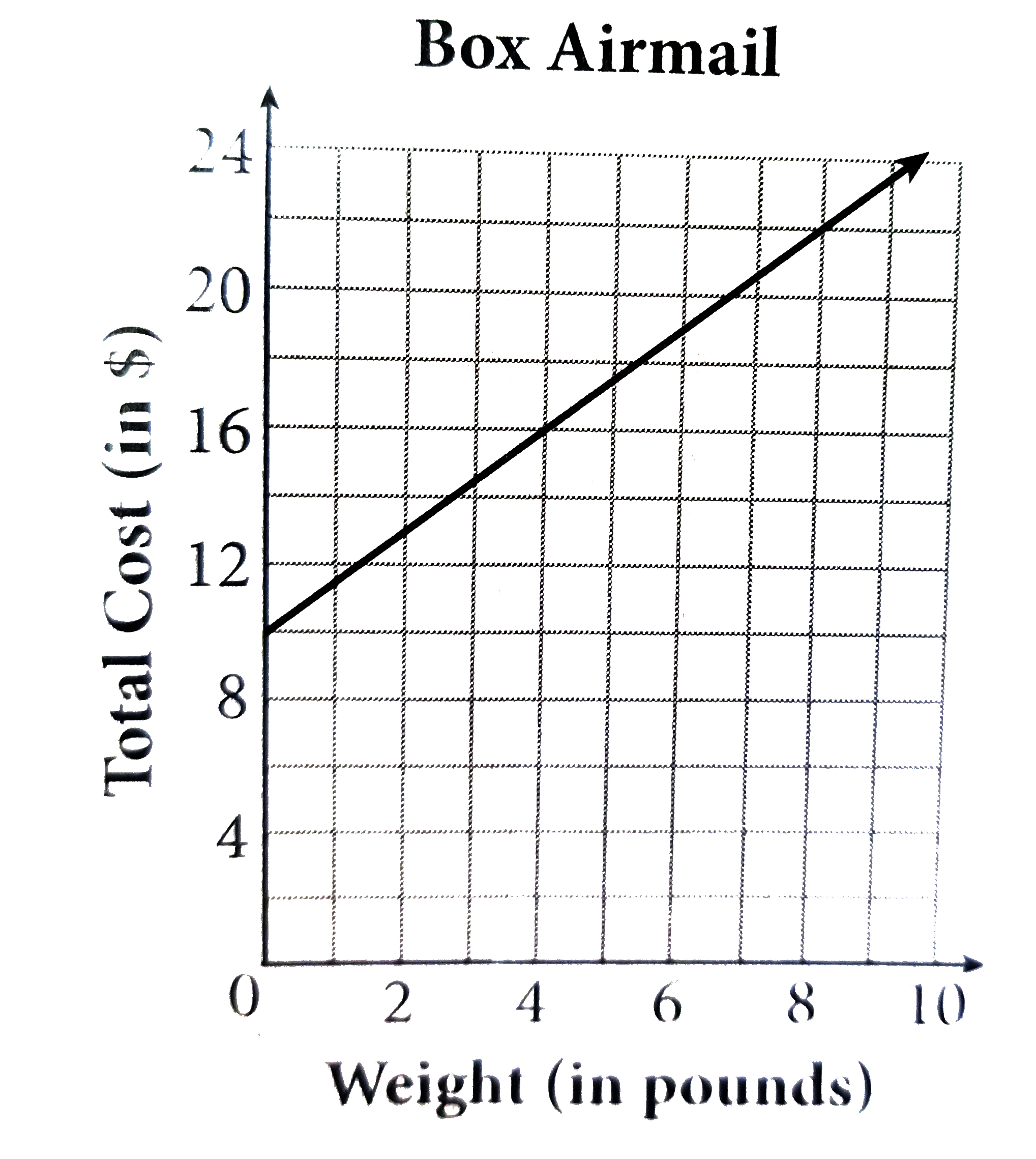#A freight airline charges a flat fee to airmil a box, plus an additional charge for each pound the box weights. The graph above shows the relationship between the weitht of the box and the total cost to airmail it. Based on the graph, how much would it cost in dollars to airmail a 40-pound boxKhareedo DN Pro and dekho sari videos bina kisi ad ki rukaavat ke!

##### Updated On: 27-06-2022

Text Solution

Solution
Because 40 poinds is not shown on the graph, you nees more information. Ln a real-world scenario, the y-intercept of a graph usually represents a flat fee or a satarting amount. The slope of the line represents a unit rate, such as the cost per poind to airmail the box.
Thte y-intercept of the graph is 10, so the flat fee is $10. To find the cost per pound (the unit rate), substitute two points from the graph into the slope formula. Using the points (0,10)and(4,16), the cost per pound is 161040=64=1.5, which means it costs$1.50 per pound to airmail a box. The total cost to airmil a 40-poind box is $10+1.50(40)=$10+$60=$70. Gird in 70.

are question isn't the graph given to us where x-axis represents the weight in lbs and y axis represents the costs $10 off a box near me and the question save dataframe you line charges for flat feet to a mailbox plus an additional charge for each found the box be the graph above shows the relationship between the weight of the box and the total cost to air mail ID based on the graph how much would it cost$10 to airmail of 40 lb box so we are given a graph of a box air mail which is charged by and Pride airline at flat feet to m l a box and an additional charge for each round box so a box vev0 found that is the fixed charge is required to mailbox is 10 to this point between 2010 and in the box weighs 2 LBS then the additional charge + the weight of the box charge
come 13 date with less than 14 and greater than 12 it is somewhat 13 so the point becomes 213 and the weight of the boxes 4lbs then it is the charge is $15 to the point becomes 416 you now we are required to calculate the cost of mailing a box in Dollars in the weight of the box is 40 Pounds so our solution will be firstly we will calculate the journal equation of the line given to us and be the general equation of the line when the two points are given to us is given by Y minus y 1 x is equal to y 2 minus one upon x 2 - X 1 x x minus x one considers 0.1 and 4.2 then distance rx1 this is a Y1 this is our next to win this is why to putting the values we get Y - Y1 is 10 which is equal to 16 - 10 upon 4 - 0 x x minus zero to this becomes Y - 10 is equal to fix upon this is the journal equation which we get off the line and we are given we are required to calculate the cost of mailing a box is the box based 40 pounds and we know that the x-axis represents the weight in lbs and y axis represents the cost in Dollars to hear the value of x is given to us as 40 and they are required to calculate the value of x is 40 and we are required to calculate why should it becomes Y - 10 in the equal to 4 X 40 then this becomes 10 5 -10 is equal to 64 Y is equal to 60 + 10 ritual$70 to this is the charge which is required to mail box of 40 pounds to \$70 the charge required to Mela 40 found this is our